## 概述#

GTSAM工具箱（GTSAM表示“Georgia Tech Smoothing and Mapping”）为BSD协议的基于因子图的C++库，由我在Georgia Institute of Technology自己、学生们和同事们。它为SLAM和SFM问题提供了最先进的解决方案，但也可用于建模和解决更简单和更复杂的估计问题。它还提供了一个MATLAB界面，可以进行快速的原型开发、可视化和用户互动。

GTSAM利用稀疏性来提高计算效率。通常情况下，测量只提供少数变量之间的关系信息，因此产生的因子图将是稀疏的连接。GTSAM中实施的算法利用了这一点，以减少计算的复杂性。即使当图太密集而无法用直接方法有效处理时，GTSAM提供的迭代方法也是相当有效的。

## 1 因子图#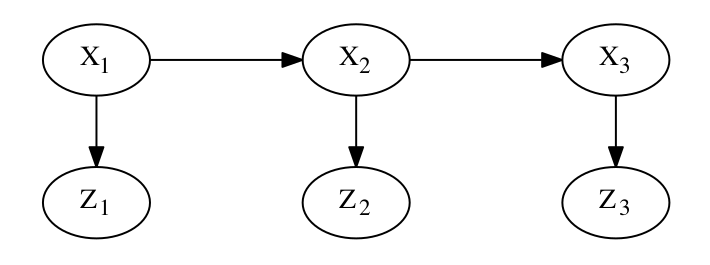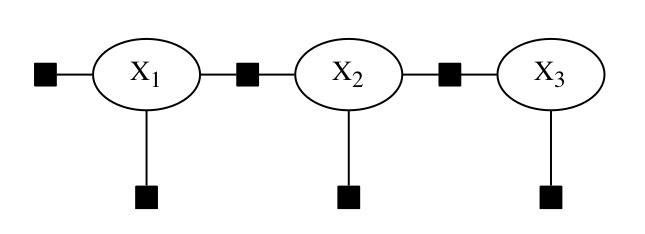## 2 对机器人运动建模#

### 2.1 使用因子图建模#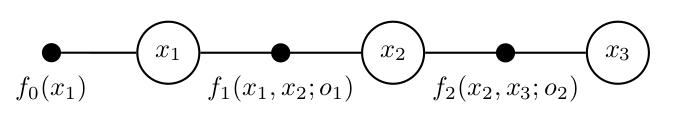### 2.2 创建因子图#

// 创建空的非线性因子图
NonlinearFactorGraph graph;

//在位置x_1位置添加高斯先验
Pose2 priorMean(0.0, 0.0, 0.0);
noiseModel::Diagonal::shared_ptr priorNoise =
noiseModel::Diagonal::Sigmas(Vector3(0.3, 0.3, 0.1));

// 添加两个里程因子
Pose2 odometry(2.0, 0.0, 0.0);
noiseModel::Diagonal::shared_ptr odometryNoise =
noiseModel::Diagonal::Sigmas(Vector3(0.2, 0.2, 0.1));
graph.print("\nFactor Graph:\n");

Factor Graph:
size: 3

Factor 0: PriorFactor on 1
prior mean:  (0, 0, 0)
noise model: diagonal sigmas[0.3; 0.3; 0.1];

Factor 1: BetweenFactor(1,2)
measured:  (2, 0, 0)
noise model: diagonal sigmas[0.2; 0.2; 0.1];

Factor 2: BetweenFactor(2,3)
measured:  (2, 0, 0)
noise model: diagonal sigmas[0.2; 0.2; 0.1];


### 2.3 因子图相对（versus）值#

1. 因子图及其在代码中的体现指定了机器人整个轨迹$$X\triangleq\{x_1 , x_2 , x_3\}$$的联合概率分布$$P(X|Z)$$，而不仅仅是最后的姿势。这种平滑的世界观给了GTSAM一个名字：“平滑和映射”。在本文的后面，我们将讨论如何使用GTSAM来做滤波（你经常不想做）或增量推导（我们一直在做）。
2. GTSAM中的因子图只是对概率密度$$P(X|Z)$$的规范，相应的FactorGraph类和它的派生类并不包含“解决方案”。相反，有一个单独的类型Values，用来指定（本例中）$$x_1$$$$x_2$$$$x_3$$的特定值，然后可以用来评估与特定值相关的概率（或者，更常见的，误差）。

### 2.4 GTSAM中的非线性优化#

// 创建（故意不准确的）初步估计
Values initial;
initial.insert(1, Pose2(0.5, 0.0, 0.2));
initial.insert(2, Pose2(2.3, 0.1, -0.2));
initial.insert(3, Pose2(4.1, 0.1, 0.1));

// 使用Levenberg-Marquardt优化法进行优化
Values result = LevenbergMarquardtOptimizer(graph, initial).optimize();
result.print("Initial Estimate");

Initial Estimate
Values with 3 values:
Value 1: (gtsam::Pose2)
(7.46978309e-16, -5.34409095e-16, -1.78381861e-16)

Value 2: (gtsam::Pose2)
(2, -1.09236636e-15, -2.48671177e-16)

Value 3: (gtsam::Pose2)
(4, -1.70076056e-15, -2.50943862e-16)

### 2.5 完全后验推断#

$f_X(x)=\frac{d}{dx}F_X(x)=\int_{-\infty}^{+\infty}f(x,y)dy$

// 查询边缘
cout.precision(2);
Marginals marginals(graph, result);
cout << "x1 covariance: \n" << marginals.marginalCovariance(1) << endl;
cout << "x2 covariance: \n" << marginals.marginalCovariance(2) << endl;
cout << "x3 covariance: \n" << marginals.marginalCovariance(3) << endl;

x1 covariance:
0.09 3.2e-33 2.8e-33
3.2e-33    0.09 2.6e-17
2.8e-33 2.6e-17    0.01
x2 covariance:
0.13 1.2e-18 6.1e-19
1.2e-18    0.17    0.02
6.1e-19    0.02    0.02
x3 covariance:
0.17 8.6e-18 2.7e-18
8.6e-18    0.37    0.06
2.7e-18    0.06    0.03

## 3 机器人定位#

### 3.1 单一的测量因素#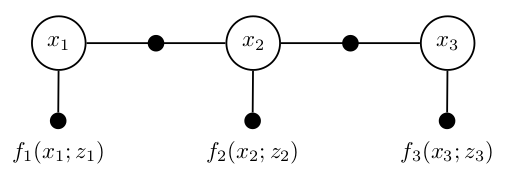### 3.2 定义自定义因子#

class UnaryFactor : public NoiseModelFactor1<Pose2>
{
double mx_, my_;        ///< X和Y测量值
public:
UnaryFactor(Key j, double x, double y, const SharedNoiseModel &model) :
NoiseModelFactor1<Pose2>(model, j), mx_(x), my_(y) {}

Vector evaluateError(const Pose2 &q,
boost::optional<Matrix &> H) const override
{
const Rot2 &R = q.rotation();
if (H) {
(*H) = (gtsam::Matrix(2, 3) <<
R.c(), -R.s(), 0.0,
R.s(), R.c(), 0.0).finished();
}
return (Vector(2) << q.x() -mx_, q.y() - my_).finished();
}
};

### 3.3 使用自定义因子#

// 添加单一测量因子，比如GPS，在所有的三个位置
auto unaryNoise = noiseModel::Diagonal::Sigmas(Vector2(0.1, 0.1));  // 10cm 标准差 on x,y
graph.add(boost::make_shared<UnaryFactor>(3, 4.0, 0.0, unaryNoise));

### 3.4 完全后验推断#

Initial Estimate
Values with 3 values:
Value 1: (gtsam::Pose2)
(1.42811204e-12, -3.93007852e-12, -4.27083461e-12)

Value 2: (gtsam::Pose2)
(2, 6.60078282e-12, 1.92677797e-13)

Value 3: (gtsam::Pose2)
(4, -2.23396394e-12, 1.90220289e-13)

x1 covariance:
0.0076 -4.6e-15  1.3e-14
-4.6e-15   0.0081  -0.0015
1.3e-14  -0.0015   0.0045
x2 covariance:
0.0071  8.5e-16 -1.3e-14
8.5e-16   0.0077  -0.0014
-1.3e-14  -0.0014   0.0072
x3 covariance:
0.0083 3.8e-15 1.1e-14
3.8e-15  0.0092  0.0026
1.1e-14  0.0026   0.017

NOTE: Python绘制结果代码如下：

import filterpy.stats as stats
import matplotlib.pyplot as plt
import numpy as np
import math

# 绘制5 Sigma椭圆，每个乘以sqrt(5)
def plot_odometry_result():
fig = plt.figure()
plt.title("Odometry")
x = np.array([7.46978309e-16, -5.34409095e-16])
P = np.array([[0.09, 3.2e-33],
[3.2e-33, 0.09]])
stats.plot_covariance(x, P * math.sqrt(5), facecolor='b', alpha=0.6)
x = np.array([2, -1.09236636e-15])
P = np.array([[0.13, 1.2e-18],
[1.2e-18, 0.17]])
stats.plot_covariance(x, P * math.sqrt(5), facecolor='b', alpha=0.6)
x = np.array([4, -1.70076056e-15])
P = np.array([[0.17, 8.6e-18],
[2.7e-18, 0.37]])
stats.plot_covariance(x, P * math.sqrt(5), facecolor='b', alpha=0.6)
plt.xlabel('x')
plt.ylabel('y')
plt.axis('equal')

def plot_localization_result():
fig = plt.figure()
plt.title("Localization")
x = np.array([1.42811204e-12, -3.93007852e-12])
P = np.array([[0.0076, -4.6e-15],
[-4.6e-15, 0.0081]])
stats.plot_covariance(x, P * math.sqrt(5), facecolor='b', alpha=0.6)
x = np.array([2, 6.60078282e-12])
P = np.array([[0.0071, 8.5e-16],
[8.5e-16, 0.0077]])
stats.plot_covariance(x, P * math.sqrt(5), facecolor='b', alpha=0.6)
x = np.array([4, -2.23396394e-12])
P = np.array([[0.0083, 3.8e-15],
[3.8e-15, 0.0092]])
stats.plot_covariance(x, P * math.sqrt(5), facecolor='b', alpha=0.6)
plt.xlabel('x')
plt.ylabel('y')
plt.axis('equal')

if __name__ == "__main__":
plot_odometry_result()
plot_localization_result()
plt.show()(a)里程计边缘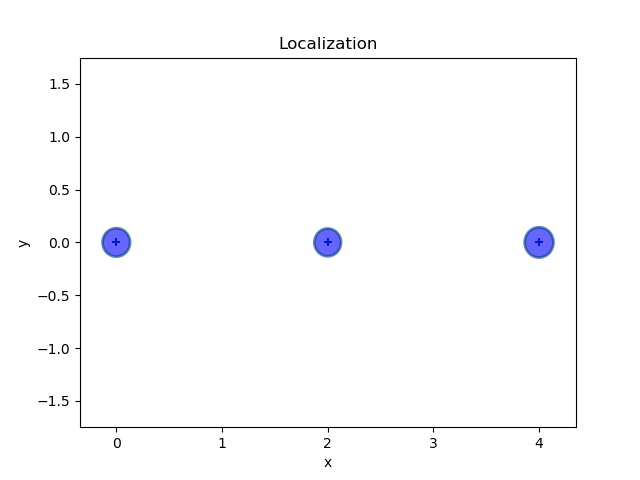(b)定位边缘